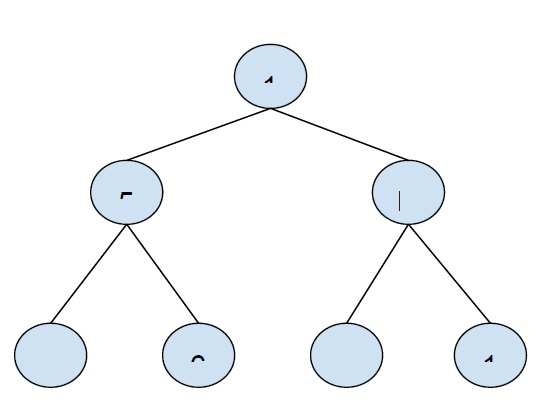# Maximum spiral sum in Binary Tree in C++

In this problem, we are given a binary tree. Our task is to create a program that will find the maximum spiral sum in a binary tree in C++.

Spiral sum of a binary tree is the sum of nodes that are encountered in the spiral traversal of the binary tree.

In the spiral traversal of a tree, the nodes are traversed from the root to the leaf node. The traversal takes place from left to right then for the next level from right to left and so on for the further levels.

ExampleOutput −5

Explanation

We will consider the spiral traversal until the first node of the 2nd level of the tree.

1+ 5 = 5.

The sum elements of the third row are (1-9+6-4 = -6) which will decrease the overall sum, hence it is eliminated while considering the maximum sum.

To solve this problem, we will be using an array that will store the sum of elements at each level, and to find the spiller sum at each level, we will be using two stacks. Then at the end, we will check if the inclusion of the sum after the level increases the maximum sum, if yes then we will take it otherwise discard the rest of the spiral.

## Example

Program to find the maximum spiral sum in the binary tree

Live Demo

#include <bits/stdc++.h>
using namespace std;
struct Node {
int data;
Node *left, *right;
};
Node* insertNode(int data){
Node* node = new Node;
node->data = data;
node->left = node->right = NULL;
return node;
}
int findMaxSum(vector<int> arr, int n){
int sum = INT_MIN;
int maxSum = INT_MIN;
for (int i = 0; i < n; i++) {
if (sum < 0)
sum = arr[i];
else
sum += arr[i];
maxSum = max(maxSum, sum);
}
return maxSum;
}
int SpiralSum(Node* root){
if (root == NULL)
return 0;
stack<Node*> sRtL;
stack<Node*> sLtR;
vector<int> arr;
sRtL.push(root);
while (!sRtL.empty() || !sLtR.empty()) {
while (!sRtL.empty()) {
Node* temp = sRtL.top();
sRtL.pop();
arr.push_back(temp->data);
if (temp->right)
sLtR.push(temp->right);
if (temp->left)
sLtR.push(temp->left);
}
while (!sLtR.empty()) {
Node* temp = sLtR.top();
sLtR.pop();
arr.push_back(temp->data);
if (temp->left)
sRtL.push(temp->left);
if (temp->right)
sRtL.push(temp->right);
}
}
return findMaxSum(arr, arr.size());
}
int main(){
Node* root = insertNode(1);
root->left = insertNode(5);
root->right = insertNode(-1);
root->left->left = insertNode(-4);
root->left->right = insertNode(6);
root->right->left = insertNode(-9);
root->right->right = insertNode(1);
cout << "Maximum Spiral Sum in binary tree : "<<SpiralSum(root);
return 0;
}

## Output

Maximum Spiral Sum in binary tree : 6